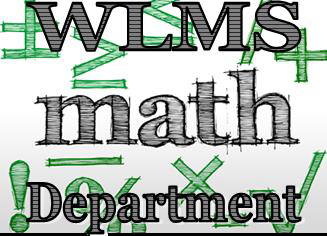## MathematicsStudents at Wolf Lake Middle get to experience learning math in an engaging, and collaborative manner. Math teachers guide students through lessons by incorporating college and career readiness standards. This student-centered, cooperative learning model teaches students to use each other, as well as their teacher, to increase their academic success. The Standards for Mathematical Practice apply throughout all courses and help to ensure that students experience mathematics as a coherent, useful, and logical subject that makes use of their ability to make sense of problem situations.

Curricular content for all subjects must integrate critical-thinking, problem-solving, and workforce-literacy skills; communication, reading, and writing skills; mathematics skills; collaboration skills; contextual and applied-learning skills; technology-literacy skills; information and media-literacy skills; and civic-engagement skills.

6th   In grade 6, instructional time will emphasize five areas: (1) performing all four operations with integers, positive decimals and positive fractions with procedural fluency; (2) exploring and applying concepts of ratios, rates and percent to solve problems; (3) creating, interpreting and using expressions and equations; (4) extending geometric reasoning to plotting points on the coordinate plane, area and volume of geometric figures and (5) extending understanding of statistical thinking.

6th Acc In grade 6 accelerated, instructional time will emphasize five areas: (1) performing all four operations with rational numbers with procedural fluency; (2) exploring and applying concepts of ratios, rates, percentages and proportions to solve problems; (3) creating, interpreting and using expressions, equations and inequalities; (4) extending geometric reasoning to plotting points on the coordinate plane, area and volume of geometric figures and (5) extending understanding of statistical thinking to represent and compare categorical and numerical data.

7th   In grade 7, instructional time will emphasize five areas: (1) recognizing that fractions, decimals and percentages are different representations of rational numbers and performing all four operations with rational numbers with procedural fluency; (2) creating equivalent expressions and solving equations and inequalities; (3) developing understanding of and applying proportional relationships in two variables; (4) extending analysis of two- and three-dimensional figures to include circles and cylinders and (5) representing and comparing categorical and numerical data and developing understanding of probability.

7th Acc  In grade 7 accelerated, instructional time will emphasize six areas: (1) representing numbers in scientific notation and extending the set of numbers to the system of real numbers, which includes irrational numbers; (2) generating equivalent numeric and algebraic expressions including using the Laws of Exponents; (3) creating and reasoning about linear relationships including modeling an association in bivariate data with a linear equation; (4) solving linear equations, inequalities and systems of linear equations; (5) developing an understanding of the concept of a function and (6) analyzing two-dimensional figures, particularly triangles, using distance, angle and applying the Pythagorean Theorem.

Pre-Algebra  In grade 8, instructional time will emphasize six areas: (1) representing numbers in scientific notation and extending the set of numbers to the system of real numbers, which includes irrational numbers; (2) generate equivalent numeric and algebraic expressions including using the Laws of Exponents; (3) creating and reasoning about linear relationships including modeling an association in bivariate data with a linear equation; (4) solving linear equations, inequalities and systems of linear equations; (5) developing an understanding of the concept of a function and (6) analyzing two-dimensional figures, particularly triangles, using distance, angle and applying the Pythagorean Theorem.

Algebra   In Algebra 1 Honors, instructional time will emphasize five areas: (1) performing operations with polynomials and radicals, and extending the Laws of Exponents to include rational exponents; (2) extending understanding of functions to linear, quadratic and exponential functions and using them to model and analyze real-world relationships; (3) solving quadratic equations in one variable and systems of linear equations and inequalities in two variables; (4) building functions, identifying their key features and representing them in various ways and (5) representing and interpreting categorical and numerical data with one and two variables.

Geometry  In Geometry Honors, instructional time will emphasize five areas: (1) proving and applying relationships and theorems involving two-dimensional figures using Euclidean geometry and coordinate geometry; (2) establishing congruence and similarity using criteria from Euclidean geometry and using rigid transformations; (3) extending knowledge of geometric measurement to two-dimensional figures and three-dimensional figures; (4) creating and applying equations of circles in the coordinate plane and (5) developing an understanding of right triangle trigonometry.